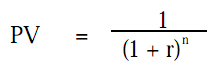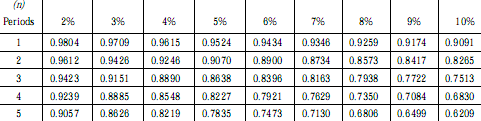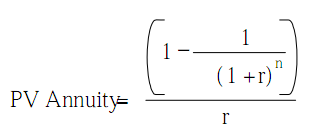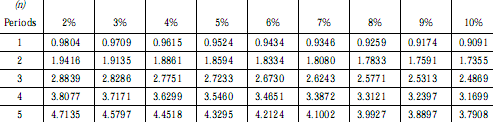Connect with us

# Estimates of Future Cash Flows and Present Value [FASB CON 7]Published

onStatement of Financial Accounting Concepts [CON] 7 provides a framework for using estimates of future cash flows as the basis for accounting measurements, either at initial recognition or when assets are subsequently re-measured at fair value (fresh-start measurements).  It also provides a framework for using the interest method of amortization.  It provides the principles that govern measurement using present value, especially when the amount of future cash flows, their timing, or both are uncertain.  However, it does not address recognition questions, such as which transactions and events should be valued using present value measures or when fresh-start measurements are appropriate.

Through this post, I describe estimates of Future cash flows and present value [FASB CON 7] in details with case examples of how to use present value formula to estimate future cash flow. Include how a risk-free analysis relates with future cash flow and as usually, a practical insight close this post. Enjoy!

Fair value is the objective for most measurements at initial recognition and for fresh-start measurements in subsequent periods.  At initial recognition, the cash paid or received (historical cost or proceeds) is usually assumed to be fair value, absent evidence to the contrary.  For fresh-start measurements, a price that is observed in the marketplace for an essentially similar asset or liability is fair value.  If purchase prices and market prices are available, there is no need to use alternative measurement techniques to approximate fair value.  However, if alternative measurement techniques must be used for initial recognition and for fresh-start measurements, those techniques should attempt to capture the elements that when taken together would comprise a market price if one existed.  The objective is to estimate the price likely to exist in the marketplace if there were a marketplace—fair value.

CON 7 states that the only objective of using present value in accounting measurements is fair value.  It is necessary to capture, to the extent possible, the economic differences in the marketplace between sets of estimated future cash flowsA present value measurement that fully captures those differences must include the following elements:

• An estimate of the future cash flow, or in more complex cases, series of future cash flows at different times
• Expectations about possible variations in the amount or timing of those cash flows
• The time value of money, represented by the risk-free rate of interest
• The risk premium—the price for bearing  the uncertainty inherent in the asset or liability
• Other factors, including illiquidity and market imperfections

### How CON 7 Measures Differ From Previously Utilized Present Value Techniques

Previously employed present value techniques typically used a single set of estimated cash flows and a single discount (interest) rate.  In applying those techniques, adjustments for factors 2 through 5 described in the previous paragraph are incorporated in the selection of the discount rate.  In the CON 7 approach, only the third factor listed (the time value of money) is included in the discount rate; the other factors cause adjustments in arriving at risk-adjusted expected cash flows.  CON 7 introduces the probability-weighted, expected cash flow approach, which focuses on the range of possible estimated cash flows and estimates of their respective probabilities of occurrence.

Previous techniques used to compute present value used estimates of the cash flows most likely to occur.  CON 7 refines and enhances the precision of this model by weighting different cash flow scenarios (regarding the amounts and timing of cash flows) by their estimated probabilities of occurrence and factoring these scenarios into the ultimate determination of fair value.  The difference is that values are assigned to the cash flows other than the most likely one.

Case Example

To illustrate, a cash flow might be \$100, \$200, or \$300 with probabilities of 10%, 50%, and 40%, respectively.  The most likely cash flow is the one with 50% probability, or \$200.  The expected cash flow is \$230 [=(\$100 × 0.1) + (\$200 × 0.5) + (\$300 × 0.4)].

The CON 7 method, unlike previous present value techniques, can also accommodate uncertainty in the timing of cash flows.  For example, a cash flow of \$10,000 may be received in one year, two years, or three years with probabilities of 15%, 60%, and 25%, respectively.  Traditional present value techniques would compute the present value using the most likely timing of the payment—two years.  The example below shows the computation of present value using the CON 7 method.  Again, the expected present value of \$9,030 differs from the traditional notion of a best estimate of \$9,070 (the 60% probability) in this example.

Present value of \$10,000 in 1 year discounted at 5%  \$9,523
Multiplied by 15% probability                                                                  \$1,428
Present value of \$10,000 in 2 years discounted at 5%  \$9,070
Multiplied by 60% probability                                                                    5,442
Present value of \$10,000 in 3 years discounted at 5%  \$8,638
Multiplied by 25% probability                                                                    2,160
Probability weighted expected present value                                           \$9,030

### Measuring Liabilities

The measurement of liabilities involves different problems from the measurement of assets; however, the underlying objective is the same.  When using present value techniques to estimate  the fair value of a liability,  the objective is to estimate the value of the assets required currently to (1) settle the liability with the holder or (2) transfer the liability to an entity of comparable credit standing

To estimate the fair value of an entity’s notes or bonds payable, accountants look to the price at which other entities are willing to hold the entity’s liabilities as assets.  For example, the proceeds of a loan are the price that a lender paid to hold the borrower’s promise of future cash flows as an asset.

The most relevant measurement of an entity’s liabilities should always reflect the credit standing of the entity.  An entity with a good credit standing will receive more cash for its promise to pay than an entity with a poor credit standing.  For example, if two entities both promise to pay \$750 in three years with no stated interest payable in the interim, Entity A, with a good credit standing, might receive about \$630 (a 6% interest rate).  Entity B, with a poor credit standing, might receive about \$533 (a 12% interest rate).  Each entity initially records its respective liability at fair value, which is the amount of proceeds received—an amount that incorporates that entity’s credit standing.

Present value techniques can also be used to value a guarantee of a liability.  Assume that Entity B in the above example owes Entity C.  If Entity A were to assume the debt, it would want to be compensated \$630—the amount that it could get in the marketplace for its promise to pay \$750 in three years.  The difference between what Entity A would want to take the place of Entity B (\$630) and the amount that Entity B receives (\$533) is the value of the guarantee (\$97).

### Interest Method of Allocation

CON 7 describes the factors that suggest that an interest method of allocation should be used.  It states that the interest method of allocation is more relevant than other methods of cost allocation when it is applied to assets and liabilities that exhibit one or more of the following characteristics:

• The transaction is, in substance, a borrowing and lending transaction.
• Period-to-period allocation of similar assets or liabilities employs an interest method.
• A particular set of estimated future cash flows is closely associated with the asset or liability.
• The measurement at initial recognition was based on present value.

### Accounting For Changes In Expected Cash Flows

If the timing or amount of estimated cash flows changes and the asset or liability is not re-measured at a fresh-start measure, the interest method of allocation should be altered by a catch-up approach.  That approach adjusts the carrying amount to the present value of the revised estimated future cash flows, discounted at the original effective interest rate.

### Application of Present Value Tables And Formulas

Present value of a single future amount – To take the present value of a single amount that will be paid in the future, apply the following formula;where:

• PV is the present value of \$1 paid in the future,
• r is the interest rate per period; and
• n” is the number of periods between the current date and the future date when the amount will be realized.

In many cases the results of this formula are summarized in a present value factor table.Case Example

Suppose one wishes to determine how much would need to be invested today to have \$10,000 in 5 years if the sum invested would earn 8%.  Looking across the row with n = 5 and finding the present value factor for the r = 8% column, the factor of 0.6806 would be identified.

Multiplying \$10,000 by 0.6806 results in \$6,806, the amount that would need to be invested today to have \$10,000 at the end of 5 years.  Alternatively, using a calculator and applying the present value of a single sum formula, one could multiply \$10,000 by the fomula, which would also give the same answer—\$6,806.

Present value of a series of equal payments (an annuity) – Many times in business situations a series of equal payments paid at equal time intervals is required.  Examples of these include payments of semiannual bond interest and principal or lease payments.  The present value of each of these payments could be added up to find the present value of this annuity, or alternatively a much simpler approach is available.  The formula for calculating the present value of an annuity of \$1 payments over “n” periodic payments, at a periodic interest rate of “r” is:The results of this formula are summarized in an annuity present value factor table.Case Example

Suppose four annual payments of \$1,000 will be needed to satisfy an agreement with a supplier.  What would be the amount of the liability today if the interest rate the supplier is charging is 6% per year?  Using the table to get the present value factor, the n = 4 periods row, and the 6% column, gives you a factor of 3.4651.  Multiply this by \$1,000 and you get a liability of \$3,465.10 that should be recorded.  Using the formula would also give you the same answer with r = 6% and n = 4.

Caution must be exercised when payments are not to be made on an annual basis.  If payments are on a semiannual basis n = 8, but r is now 3%.  This is because r is the periodic interest rate, and the semiannual rate would not be 6%, but half of the 6% annual rate.  Note that this is somewhat simplified, since due to the effect of compound interest 3% semiannually is slightly more than a 6% annual rate.

### Example of The Relevance of Present Values

A measurement based on the present value of estimated future cash flows provides more relevant information than a measurement based on the undiscounted sum of those cash flows

For example: consider the following four future cash flows, all of which have an undiscounted value of \$100,000:

• Asset A has a fixed contractual cash flow of \$100,000 due tomorrow.  The cash flow is certain of receipt.
• Asset B has a fixed contractual cash flow of \$100,000 due in twenty years.  The cash flow is certain of receipt.
• Asset C has a fixed contractual cash flow of \$100,000 due in twenty years.  The amount that ultimately will be received is uncertain.  There is an 80% probability that the entire \$100,000 will be received.  There is a 20% probability that \$80,000 will be received.
• Asset D has an expected cash flow of \$100,000 due in twenty years.  The amount that ultimately will be received is uncertain.  There is a 25% probability that \$120,000 will be received.   There is a 50% probability that \$100,000 will be received.  There is a 25% probability that \$80,000 will be received.

Assuming a 5% risk-free rate of return, the present values of the assets are:

• Asset A has a present value of \$99,986.  The time value of money assigned to the one-day period is \$14 [\$100,000 × 0.05/365 days]
• Asset B has a present value of \$37,689 [\$100,000/(1 + 0.05)20]
• Asset C has a present value of \$36,181 [(100,000 × 0.8 + 80,000 × 0.2)/(1 + .05)20]
• Asset D has a present value of \$37,689 [(\$120,000 × 0.25 + 100,000 × 0.5 + 80,000 ×
0.25)/(1 + 0.05)20]

Although each of these assets has the same undiscounted cash flows, few would argue that they are economically the same or that a rational investor would pay the same price for each.  Investors require compensation for the time value of money.  They also require a risk premium.  That is, given a choice between Asset B with expected cash flows that are certain and Asset D with cash flows of  the same expected amount that are uncertain, investors will place a higher value on Asset B,  even though they have the same expected present value.

CON 7 says that the risk premium should be subtracted from the expected cash flows before applying the discount rate.  Thus, if the risk premium for Asset D was \$500, the risk-adjusted present values would be \$37,500 {[(\$120,000 × .25 + 100,000 × .5 + 80,000 × .25) – 500]/ (1 + .05)20}.

### Practical Insight: The Application of Expected Cash Flow Approach

Like any accounting measurement, the application of an expected cash flow approach is subject to a cost-benefit constraint.  The cost of obtaining additional information must be weighed against the additional reliability that information will bring to the measurement.  As a practical matter, an entity that uses present value measurements often has little or no information about some or all of the assumptions that investors would use in assessing the fair value of an asset or a liability.  Instead, the entity must use the information that is available to it without undue cost and effort when it develops cash flow estimates.

The entity’s own assumptions about future cash flows can be used to estimate fair value using present value techniques, as long as there are no contrary data indicating that investors would use different assumptions.  However, if contrary data exist, the entity must adjust its assumptions to incorporate that market information.

Are you looking for easy accounting tutorial? Established since 2007, Accounting-Financial-Tax.com hosts more than 1300 articles (still growing), and has helped millions accounting student, teacher, junior accountants and small business owners, worldwide.